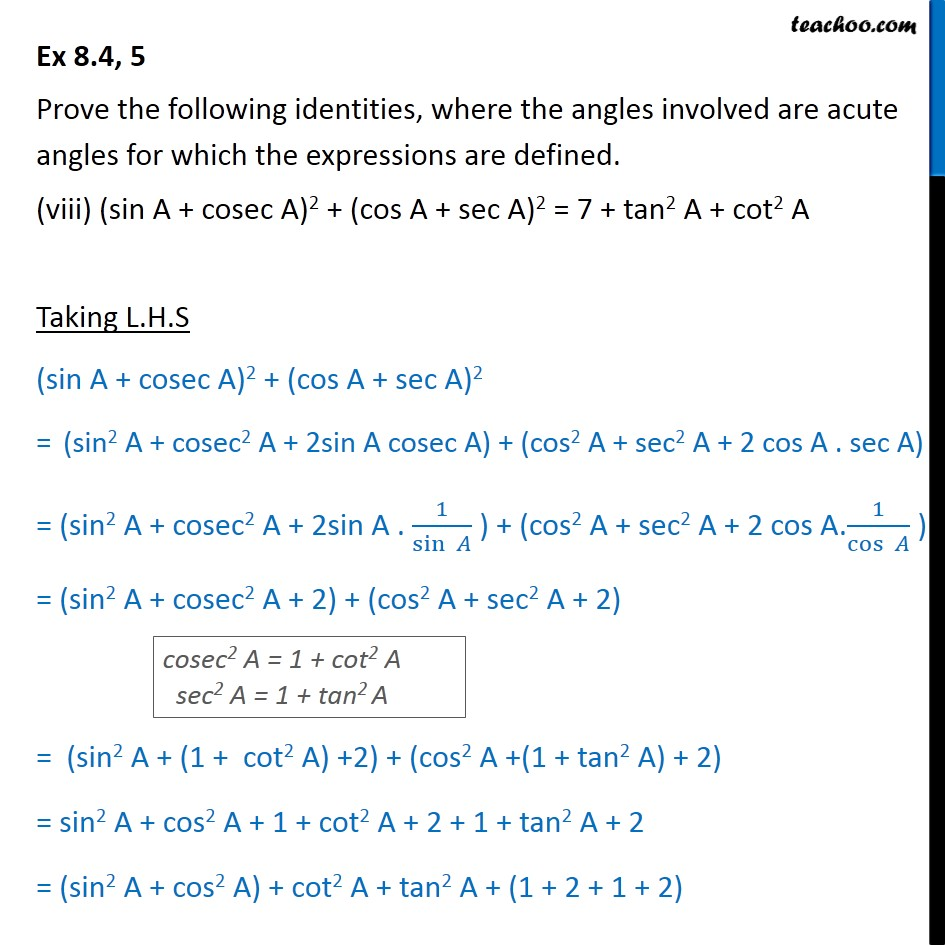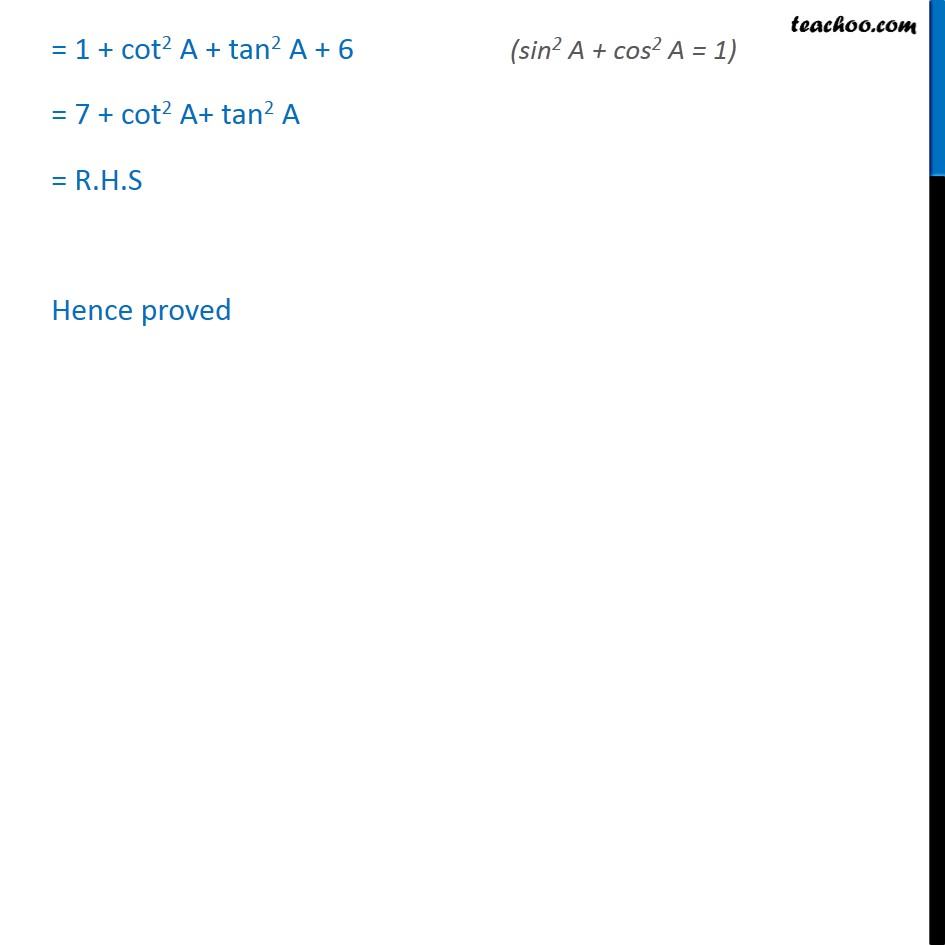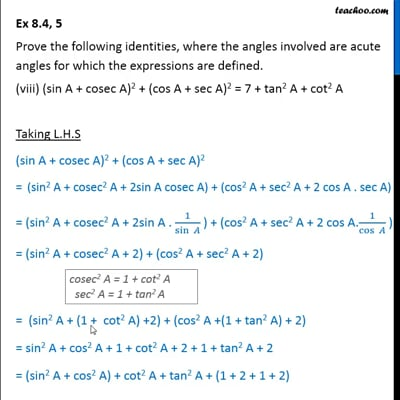Ex 8.4

Chapter 8 Class 10 Introduction to Trignometry
Serial order wiseThis video is only available for Teachoo black users

Introducing your new favourite teacher - Teachoo Black, at only ₹83 per month

### Transcript

Ex 8.4, 5 Prove the following identities, where the angles involved are acute angles for which the expressions are defined. (sin A + cosec A)2 + (cos A + sec A)2 = 7 + tan2 A + cot2 A Taking L.H.S (sin A + cosec A)2 + (cos A + sec A)2 = (sin2 A + cosec2 A + 2sin A cosec A) + (cos2 A + sec2 A + 2 cos A . sec A) = (sin2 A + cosec2 A + 2sin A . 1/sin ) + (cos2 A + sec2 A + 2 cos A.1/cos ) = (sin2 A + cosec2 A + 2) + (cos2 A + sec2 A + 2) = (sin2 A + (1 + cot2 A) +2) + (cos2 A +(1 + tan2 A) + 2) = sin2 A + cos2 A + 1 + cot2 A + 2 + 1 + tan2 A + 2 = (sin2 A + cos2 A) + cot2 A + tan2 A + (1 + 2 + 1 + 2) = 1 + cot2 A + tan2 A + 6 = 7 + cot2 A+ tan2 A = R.H.S Hence proved